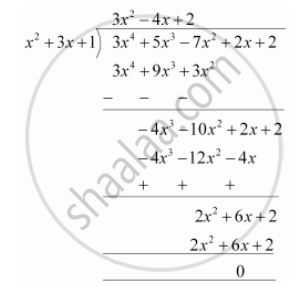Share

Books Shortlist

# Check whether the first polynomial is a factor of the second polynomial by dividing the second polynomial by the first polynomial  x^2 + 3x + 1, 3x^4 + 5x^3 – 7x^2 + 2x + 2 - CBSE Class 10 - Mathematics

ConceptDivision Algorithm for Polynomials

#### Question

Check whether the first polynomial is a factor of the second polynomial by dividing the second polynomial by the first polynomial

x2 + 3x + 1, 3x4 + 5x3 – 7x2 + 2x + 2

#### Solution

x2 + 3x + 1, 3x4 + 5x3 – 7x2 + 2x + 2Since the remainder is 0

hence x+ 3x +1 is a factor of 3x4 + 5x3 - 7x2 + 2x + 2

Is there an error in this question or solution?

#### APPEARS IN

NCERT Solution for Mathematics Textbook for Class 10 (2019 to Current)
Chapter 2: Polynomials
Ex.2.30 | Q: 2.2 | Page no. 36

#### Video TutorialsVIEW ALL 

Solution Check whether the first polynomial is a factor of the second polynomial by dividing the second polynomial by the first polynomial  x^2 + 3x + 1, 3x^4 + 5x^3 – 7x^2 + 2x + 2 Concept: Division Algorithm for Polynomials.
S